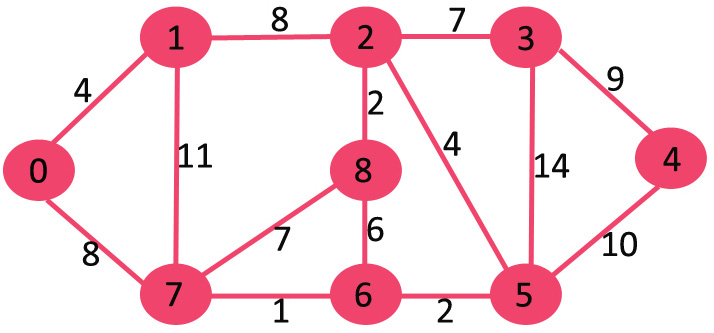Related Articles

# Dijkstra’s shortest path algorithm using set in STL

• Difficulty Level : Medium
• Last Updated : 09 Jul, 2021

Given a graph and a source vertex in graph, find shortest paths from source to all vertices in the given graph.```Input : Source = 0
Output :
Vertex   Distance from Source
0                0
1                4
2                12
3                19
4                21
5                11
6                9
7                8
8                14```

We have discussed Dijkstra’s shortest Path implementations.

The second implementation is time complexity wise better, but is really complex as we have implemented our own priority queue. STL provides priority_queue, but the provided priority queue doesn’t support decrease key and delete operations. And in Dijkstra’s algorithm, we need a priority queue and below operations on priority queue :

• ExtractMin : from all those vertices whose shortest distance is not yet found, we need to get vertex with minimum distance.
• DecreaseKey : After extracting vertex we need to update distance of its adjacent vertices, and if new distance is smaller, then update that in data structure.

Above operations can be easily implemented by set data structure of c++ STL, set keeps all its keys in sorted order so minimum distant vertex will always be at beginning, we can extract it from there, which is the ExtractMin operation and update other adjacent vertex accordingly if any vetex’s distance becomes smaller then delete its previous entry and insert new updated entry which is DecreaseKey operation.

Below is algorithm based on set data structure.

```1) Initialize distances of all vertices as infinite.

2) Create an empty set.  Every item of set is a pair
(weight, vertex). Weight (or distance) is used used
as first item  of pair as first item is by default
used to compare two pairs.

3) Insert source vertex into the set and make its
distance as 0.

4) While Set doesn't become empty, do following
a) Extract minimum distance vertex from Set.
Let the extracted vertex be u.
b) Loop through all adjacent of u and do
following for every vertex v.

// If there is a shorter path to v
// through u.
If dist[v] > dist[u] + weight(u, v)

(i) Update distance of v, i.e., do
dist[v] = dist[u] + weight(u, v)
(i) If v is in set, update its distance
in set by removing it first, then
inserting with new distance
(ii) If v is not in set, then insert
it in set with new distance

5) Print distance array dist[] to print all shortest
paths. ```

Below is C++ implementation of above idea.

## C++

 `// Program to find Dijkstra's shortest path using STL set``#include``using` `namespace` `std;``# define INF 0x3f3f3f3f` `// This class represents a directed graph using``// adjacency list representation``class` `Graph``{``    ``int` `V;    ``// No. of vertices` `    ``// In a weighted graph, we need to store vertex``    ``// and weight pair for every edge``    ``list< pair<``int``, ``int``> > *adj;` `public``:``    ``Graph(``int` `V);  ``// Constructor` `    ``// function to add an edge to graph``    ``void` `addEdge(``int` `u, ``int` `v, ``int` `w);` `    ``// prints shortest path from s``    ``void` `shortestPath(``int` `s);``};` `// Allocates memory for adjacency list``Graph::Graph(``int` `V)``{``    ``this``->V = V;``    ``adj = ``new` `list< pair<``int``, ``int``> >[V];``}` `void` `Graph::addEdge(``int` `u, ``int` `v, ``int` `w)``{``    ``adj[u].push_back(make_pair(v, w));``    ``adj[v].push_back(make_pair(u, w));``}` `// Prints shortest paths from src to all other vertices``void` `Graph::shortestPath(``int` `src)``{``    ``// Create a set to store vertices that are being``    ``// processed``    ``set< pair<``int``, ``int``> > setds;` `    ``// Create a vector for distances and initialize all``    ``// distances as infinite (INF)``    ``vector<``int``> dist(V, INF);` `    ``// Insert source itself in Set and initialize its``    ``// distance as 0.``    ``setds.insert(make_pair(0, src));``    ``dist[src] = 0;` `    ``/* Looping till all shortest distance are finalized``       ``then setds will become empty    */``    ``while` `(!setds.empty())``    ``{``        ``// The first vertex in Set is the minimum distance``        ``// vertex, extract it from set.``        ``pair<``int``, ``int``> tmp = *(setds.begin());``        ``setds.erase(setds.begin());` `        ``// vertex label is stored in second of pair (it``        ``// has to be done this way to keep the vertices``        ``// sorted distance (distance must be first item``        ``// in pair)``        ``int` `u = tmp.second;` `        ``// 'i' is used to get all adjacent vertices of a vertex``        ``list< pair<``int``, ``int``> >::iterator i;``        ``for` `(i = adj[u].begin(); i != adj[u].end(); ++i)``        ``{``            ``// Get vertex label and weight of current adjacent``            ``// of u.``            ``int` `v = (*i).first;``            ``int` `weight = (*i).second;` `            ``//    If there is shorter path to v through u.``            ``if` `(dist[v] > dist[u] + weight)``            ``{``                ``/*  If distance of v is not INF then it must be in``                    ``our set, so removing it and inserting again``                    ``with updated less distance. ``                    ``Note : We extract only those vertices from Set``                    ``for which distance is finalized. So for them,``                    ``we would never reach here.  */``                ``if` `(dist[v] != INF)``                    ``setds.erase(setds.find(make_pair(dist[v], v)));` `                ``// Updating distance of v``                ``dist[v] = dist[u] + weight;``                ``setds.insert(make_pair(dist[v], v));``            ``}``        ``}``    ``}` `    ``// Print shortest distances stored in dist[]``    ``printf``(``"Vertex   Distance from Source\n"``);``    ``for` `(``int` `i = 0; i < V; ++i)``        ``printf``(``"%d \t\t %d\n"``, i, dist[i]);``}` `// Driver program to test methods of graph class``int` `main()``{``    ``// create the graph given in above fugure``    ``int` `V = 9;``    ``Graph g(V);` `    ``//    making above shown graph``    ``g.addEdge(0, 1, 4);``    ``g.addEdge(0, 7, 8);``    ``g.addEdge(1, 2, 8);``    ``g.addEdge(1, 7, 11);``    ``g.addEdge(2, 3, 7);``    ``g.addEdge(2, 8, 2);``    ``g.addEdge(2, 5, 4);``    ``g.addEdge(3, 4, 9);``    ``g.addEdge(3, 5, 14);``    ``g.addEdge(4, 5, 10);``    ``g.addEdge(5, 6, 2);``    ``g.addEdge(6, 7, 1);``    ``g.addEdge(6, 8, 6);``    ``g.addEdge(7, 8, 7);` `    ``g.shortestPath(0);` `    ``return` `0;``}`

Output :

```Vertex   Distance from Source
0          0
1          4
2          12
3          19
4          21
5          11
6          9
7          8
8          14```

Time Complexity: Set in C++ are typically implemented using Self-balancing binary search trees. Therefore, time complexity of set operations like insert, delete is logarithmic and time complexity of above solution is O(ELogV)).
Dijkstra’s Shortest Path Algorithm using priority_queue of STL